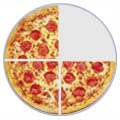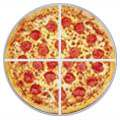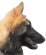# Mixed Fractions

(Also called "Mixed Numbers")134 (one and three-quarters)

A Mixed Fraction is a whole number and a proper fraction combined.

Such as 134

## Examples

 238 714 11415 2145

See how each example is made up of a whole number and a proper fraction together? That is why it is called a "mixed" fraction (or mixed number).

## Names

We can give names to every part of a mixed fraction:## Three Types of Fractions

There are three types of fraction:## Mixed Fractions or Improper Fractions

We can use either an improper fraction or a mixed fraction to show the same amount.

For example 134 = 74, as shown here:

 134 74=## Converting Improper Fractions to Mixed Fractions

To convert an improper fraction to a mixed fraction, follow these steps:Divide the numerator by the denominator. Write down the whole number answer Then write down any remainder above the denominator.

### Example: Convert 114 to a mixed fraction.

Divide:

11 ÷ 4 = 2 with a remainder of 3

Write down the 2 and then write down the remainder (3) above the denominator (4).

Answer:

2 34

That example can be written like this:### Example: Convert 103 to a mixed fraction.Answer:

3 13

## Converting Mixed Fractions to Improper Fractions

To convert a mixed fraction to an improper fraction, follow these steps:Multiply the whole number part by the fraction's denominator. Add that to the numerator Then write the result on top of the denominator.

### Example: Convert 325 to an improper fraction.

Multiply the whole number part by the denominator:

3 × 5 = 15

Add that to the numerator:

15 + 2 = 17

Then write that result above the denominator:

175

We can do the numerator in one go:### Example: Convert 219 to an improper fraction.## Are Improper Fractions Bad ?

NO, they aren't bad!

For mathematics they are actually better than mixed fractions. Because mixed fractions can be confusing when we write them in a formula: should the two parts be added or multiplied?

 Mixed Fraction: Improper Fraction: What is: 1 + 214 ? Is it: 1 + 2 + 14 = 314 ? Or is it: 1 + 2 × 14 = 112 ? What is: 1 + 94 ? It is: 44 + 94 = 134But, for everyday use, people understand mixed fractions better.

Example: It is easier to say "I ate 214 sausages", than "I ate 94 sausages"

We Recommend:

• For Mathematics: Improper Fractions
• For Everyday Use: Mixed Fractions